# 2. Evaluate the line integral fxydx + xºy’dy where C is the triangle with vertices с...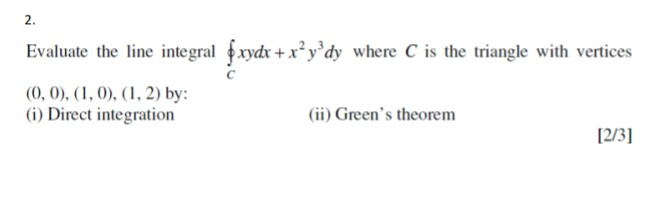2. Evaluate the line integral fxydx + xºy’dy where C is the triangle with vertices с (0, 0), (1, 0), (1, 2) by: (1) Direct integration (ii) Green's theorem [2/3]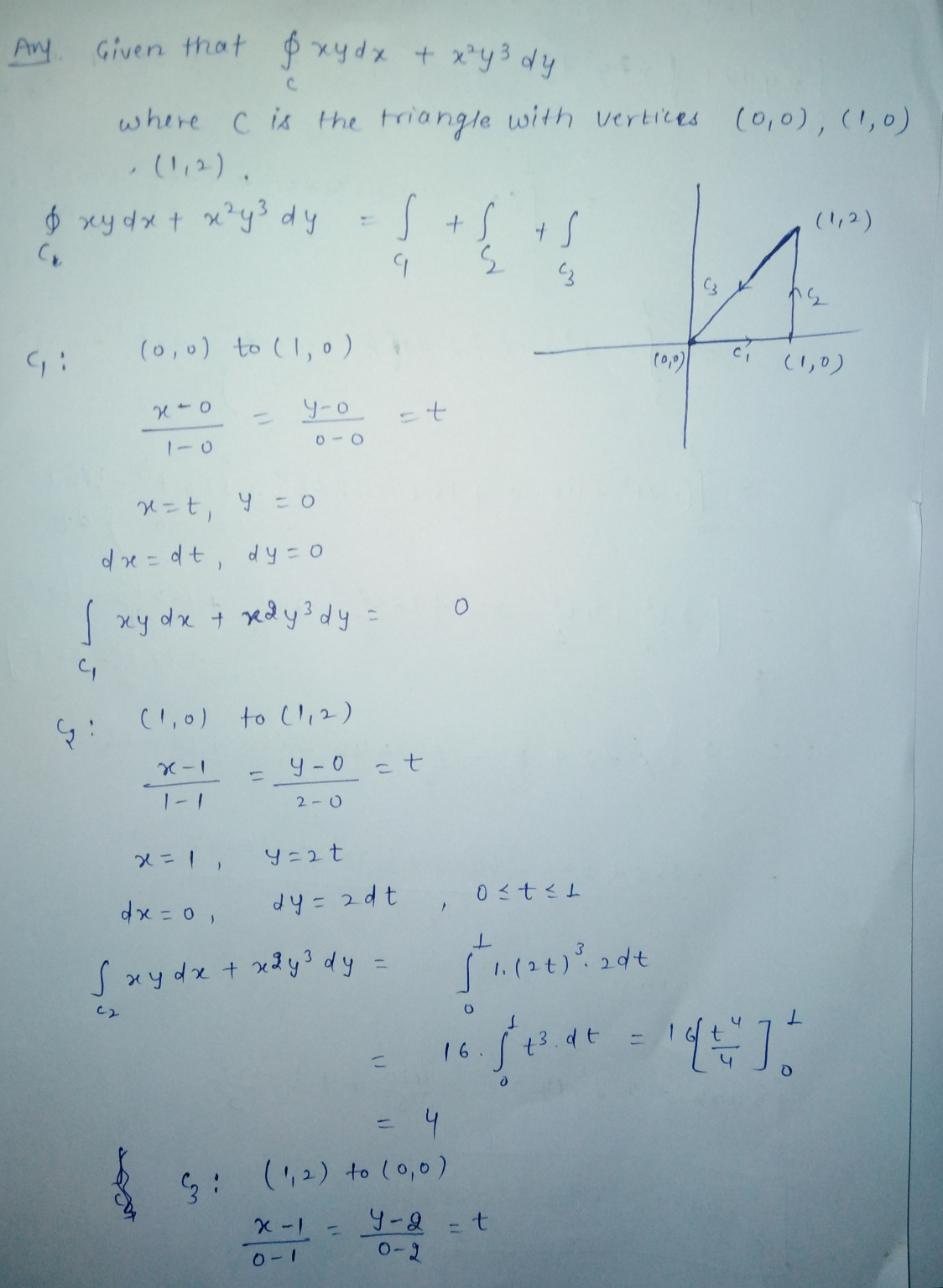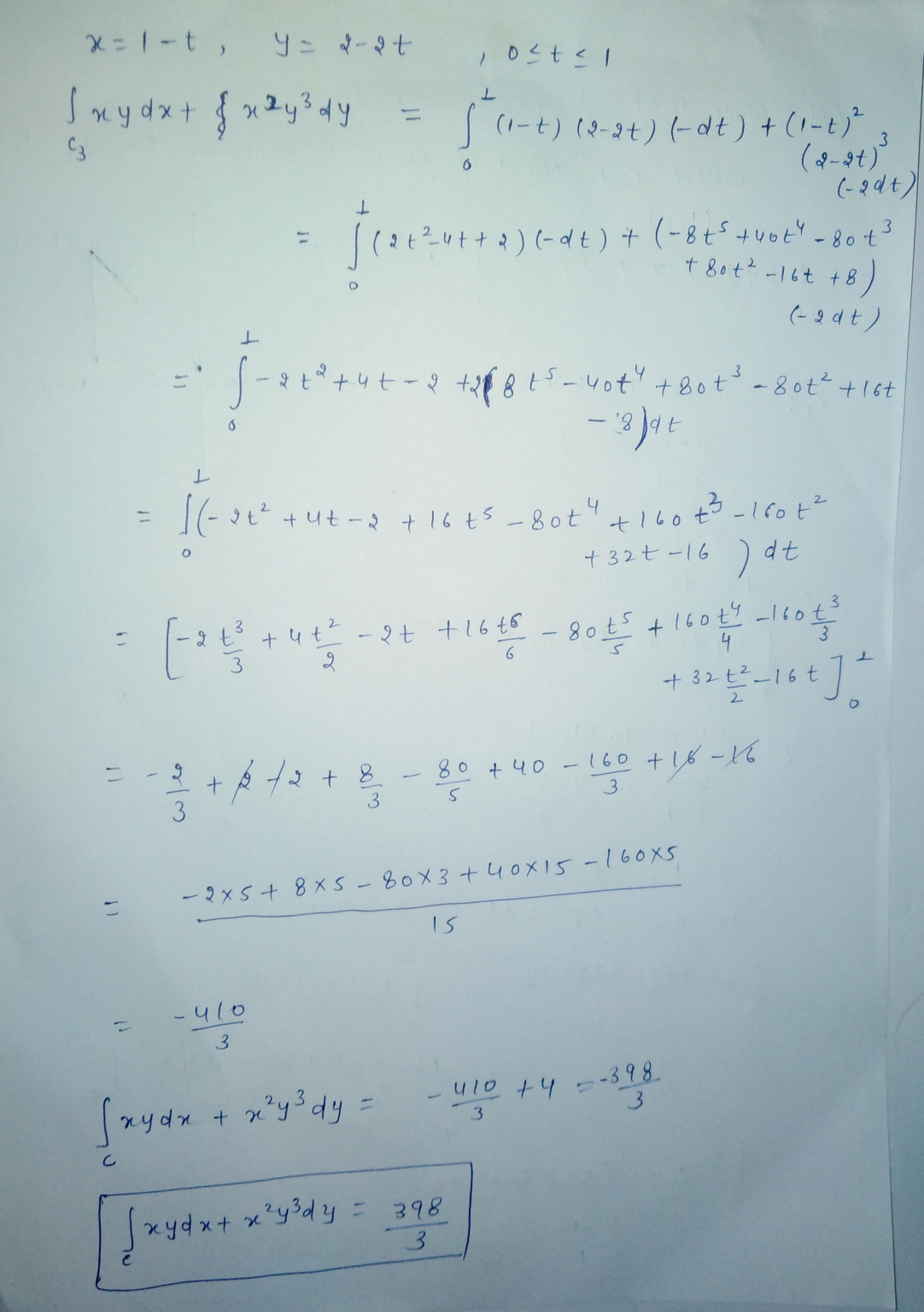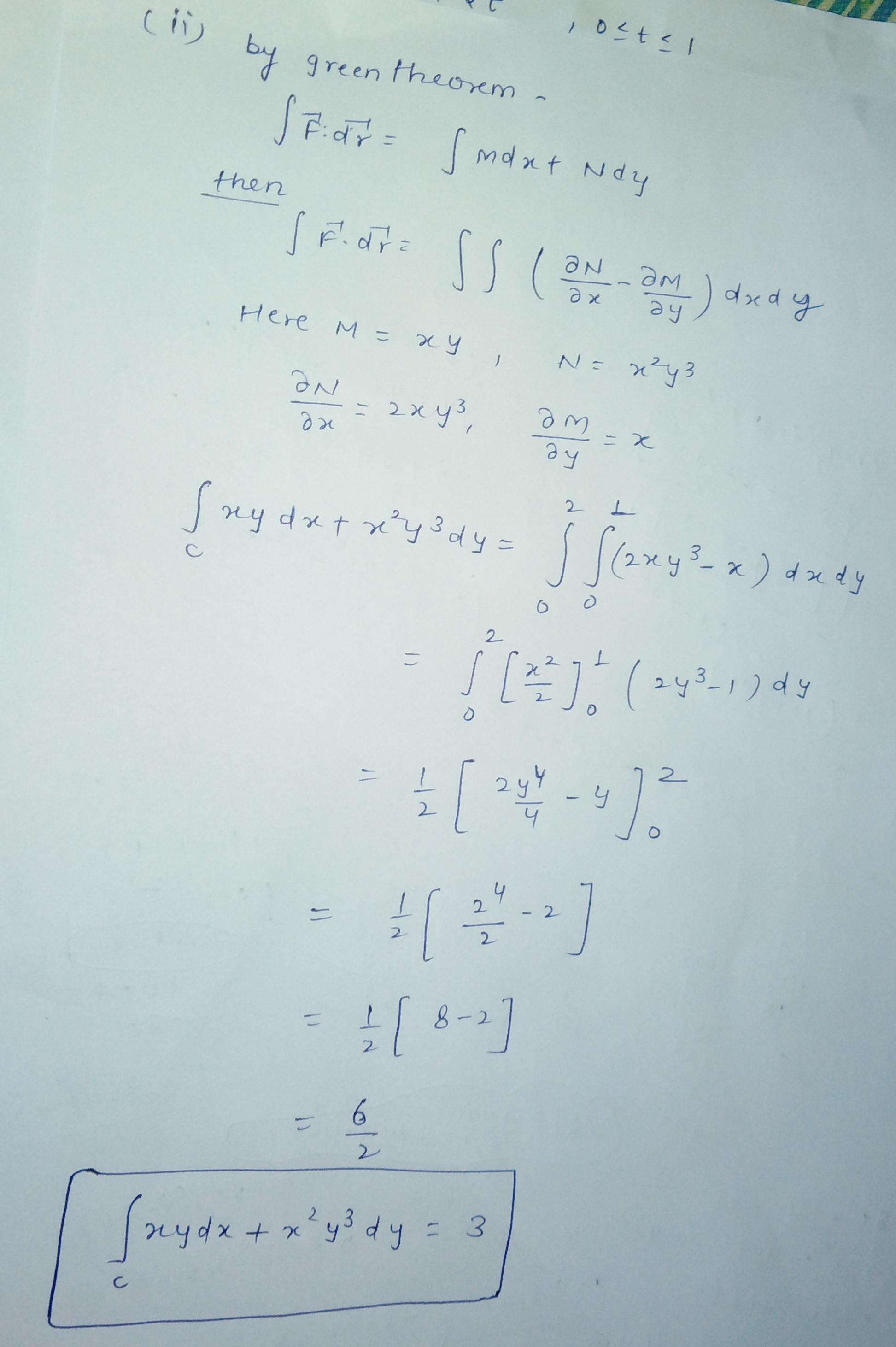##### Add Answer of: 2. Evaluate the line integral fxydx + xºy’dy where C is the triangle with vertices с...
Similar Homework Help Questions
• ### Use Green's theorem to evaluate the line integral Sc xay dx + 2xy?dx where C is...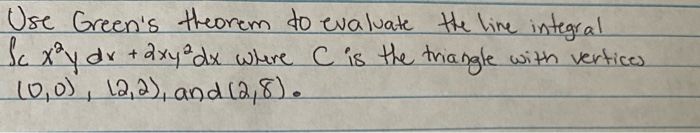Use Green's theorem to evaluate the line integral Sc xay dx + 2xy?dx where C is the triangle with vertices 10,0), 12, 2), and 12,8).

• ### Use Green's Theorem to evaluate the line integral 2xy dx + (2x + y) dy с...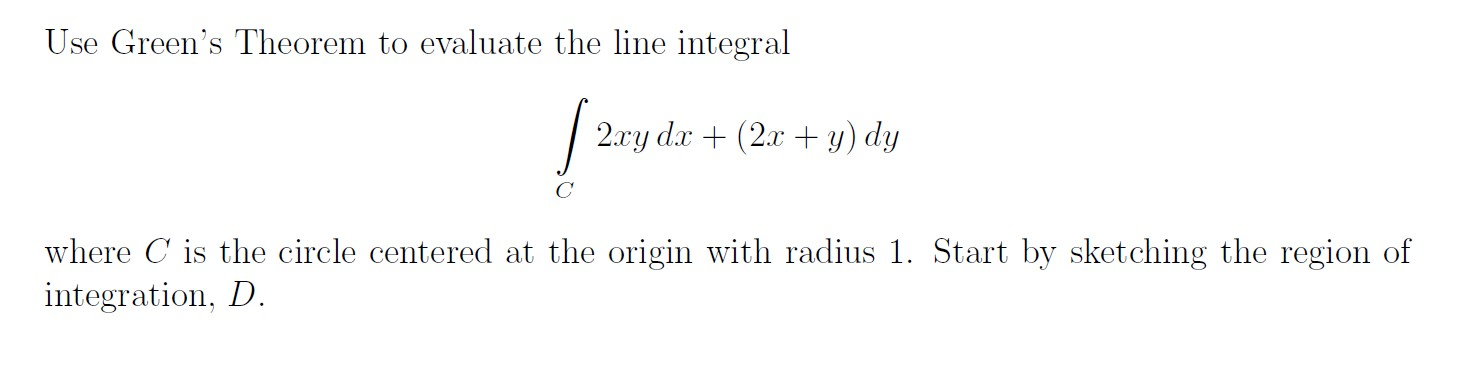Use Green's Theorem to evaluate the line integral 2xy dx + (2x + y) dy с where C is the circle centered at the origin with radius 1. Start by sketching the region of integration, D.

• ### 12. (5 Points) Use Green's Theorem to evaluate the line integral dr +(7x + cos(y?)) dy,...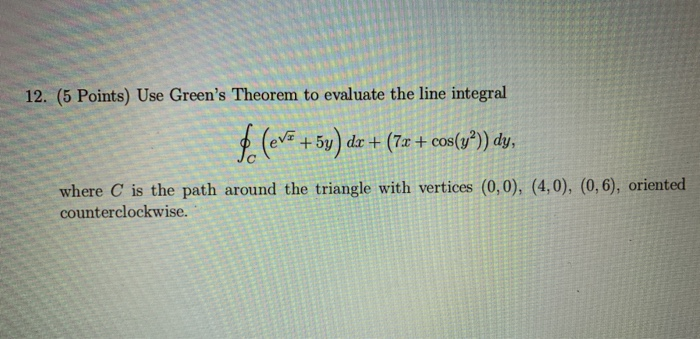12. (5 Points) Use Green's Theorem to evaluate the line integral dr +(7x + cos(y?)) dy, +5y where C is the path around the triangle with vertices (0,0), (4,0), (0,6), oriented counterclockwise. 12. (5 Points) Use Green's Theorem to evaluate the line integral dr +(7x + cos(y?)) dy, +5y where C is the path around the triangle with vertices (0,0), (4,0), (0,6), oriented counterclockwise.

• ### (a) (15 F-(1+9) 9. points) Apply Green's theorem to evaluate φ F.nds, where (x2 +y)j, of a triangle with vertices (1,0), (0,1). (-1,0) oriented in the counterclockwise direction n is the outward-...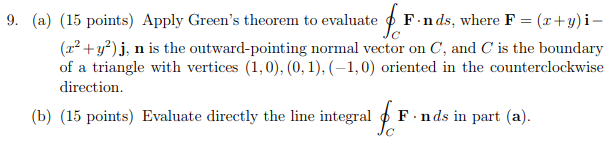(a) (15 F-(1+9) 9. points) Apply Green's theorem to evaluate φ F.nds, where (x2 +y)j, of a triangle with vertices (1,0), (0,1). (-1,0) oriented in the counterclockwise direction n is the outward-pointing normal vector on , and C is the boundary (b) (15 points) Evaluate directly the line integral p F- nds in part (a). (a) (15 F-(1+9) 9. points) Apply Green's theorem to evaluate φ F.nds, where (x2 +y)j, of a triangle with vertices (1,0), (0,1). (-1,0) oriented in...

• ### Given the following vectors F=[y2, x2,x-z] and surface S: the triangle surface with vertices (0,0...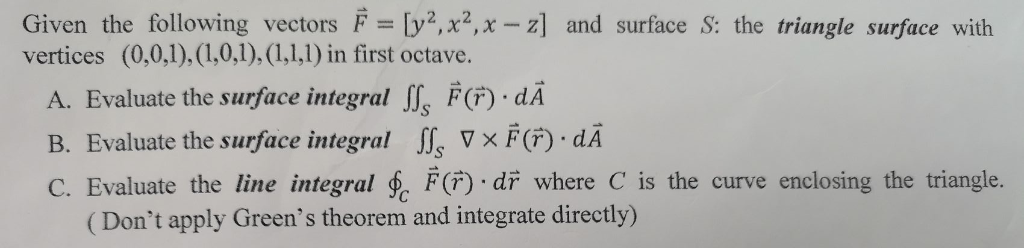Given the following vectors F=[y2, x2,x-z] and surface S: the triangle surface with vertices (0,0,1), (1,0,1), (1,1,1) in first octave. A. Evaluate the surface integral F(F) . dA B. Evaluate the surface integral VxF(F) dA C. Evaluate the line integral F() di where C is the curve enclosing the triangle. (Don't apply Green's theorem and integrate directly) Given the following vectors F=[y2, x2,x-z] and surface S: the triangle surface with vertices (0,0,1), (1,0,1), (1,1,1) in first octave. A. Evaluate the...

• ### 2. (8 pts) Use Green's Theorem to evaluate fcln(1 + y) dx - triangle with vertices...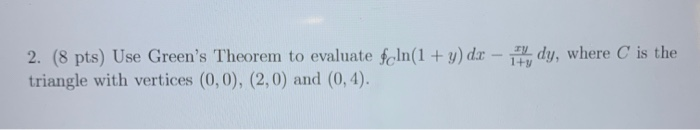2. (8 pts) Use Green's Theorem to evaluate fcln(1 + y) dx - triangle with vertices (0,0), (2,0) and (0,4). 17, dy, where C is the

• ### 6. (1 point) Use Stokes' Theorem to find the line integral /2y dx + dy + (4-3x) dz, where C is the boundary of the triangle with vertices (0,0,0), (1,3,-2), and -2,4,5), oriented counterclockwise...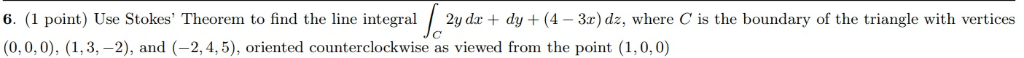6. (1 point) Use Stokes' Theorem to find the line integral /2y dx + dy + (4-3x) dz, where C is the boundary of the triangle with vertices (0,0,0), (1,3,-2), and -2,4,5), oriented counterclockwise as viewed from the point (1, 0, 0) 6. (1 point) Use Stokes' Theorem to find the line integral /2y dx + dy + (4-3x) dz, where C is the boundary of the triangle with vertices (0,0,0), (1,3,-2), and -2,4,5), oriented counterclockwise as viewed from the...

• ### please answer all 3 questions, I need help. thank you Use Green's Theorem to evaluate the...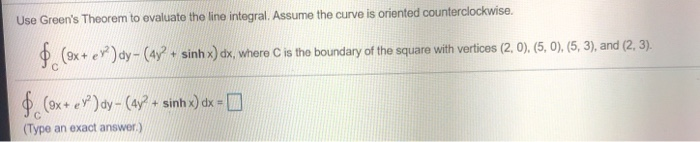please answer all 3 questions, I need help. thank you Use Green's Theorem to evaluate the line integral. Assume the curve is oriented counterclockwise. \$(9x+ ex) dy- (4y + sinh x) dx, where C is the boundary of the square with vertices (2, 0), (5, 0), (5, 3), and (2, 3). \$(9x+ ey?) ay- (4y+ + sinhx) dx = 0 (Type an exact answer.) Use Green's Theorem to evaluate the following line integral. i dy - g dx, where (19)...

• ### Evaluate the line integral by two methods: (a) directly and (b) using Green's Theorem(a) C is the circle with center the origin and radius 1(b) (thereshould be a C under (b)'s integral sign, showing that it is a curve)C is the triangle with vertices (0,0), (1,3), and (0,3)(Only use Green's Theorem to evaluate this line integral along the given positively oriented curve)

• ### Green's Theorem to Evaluate a Line IntegralUse Green's Theorem to evaluate the line integral along the given positively oriented curve.C is the rectangle with vertices (0,0), (5,0), (5,2), and (0,2)

Free Homework App